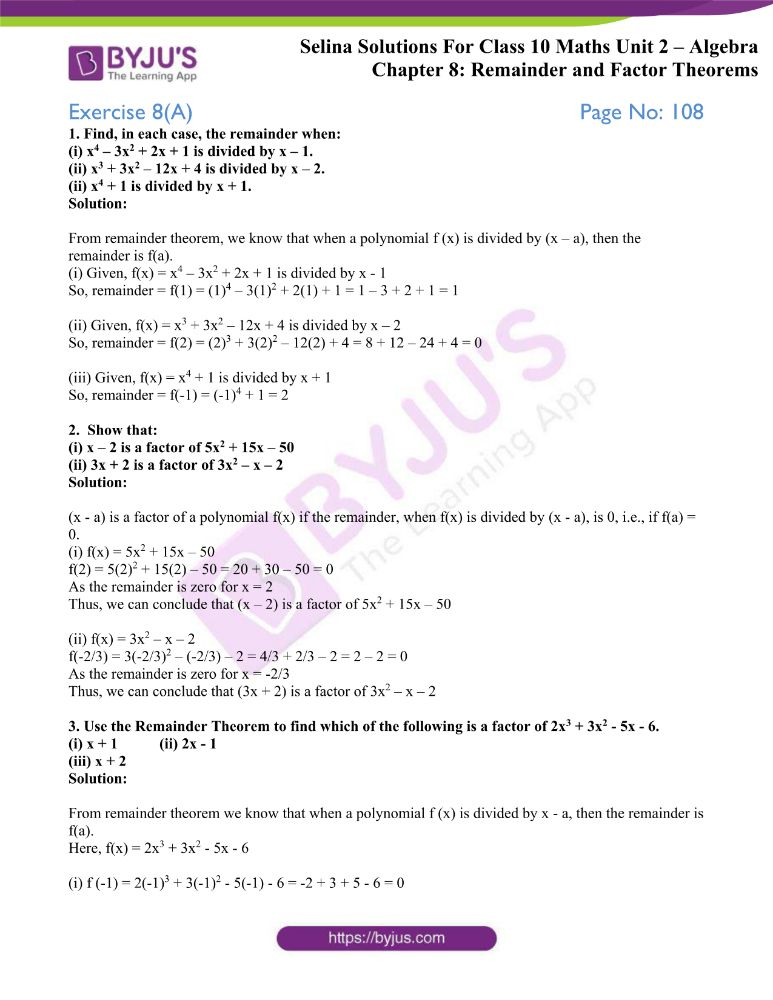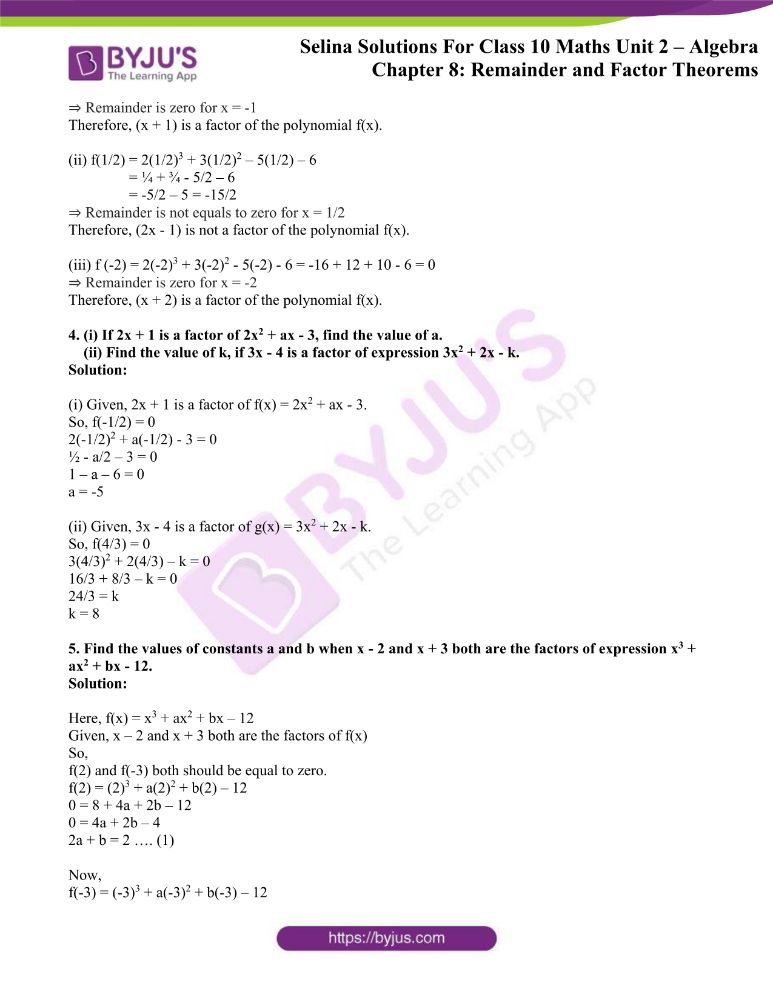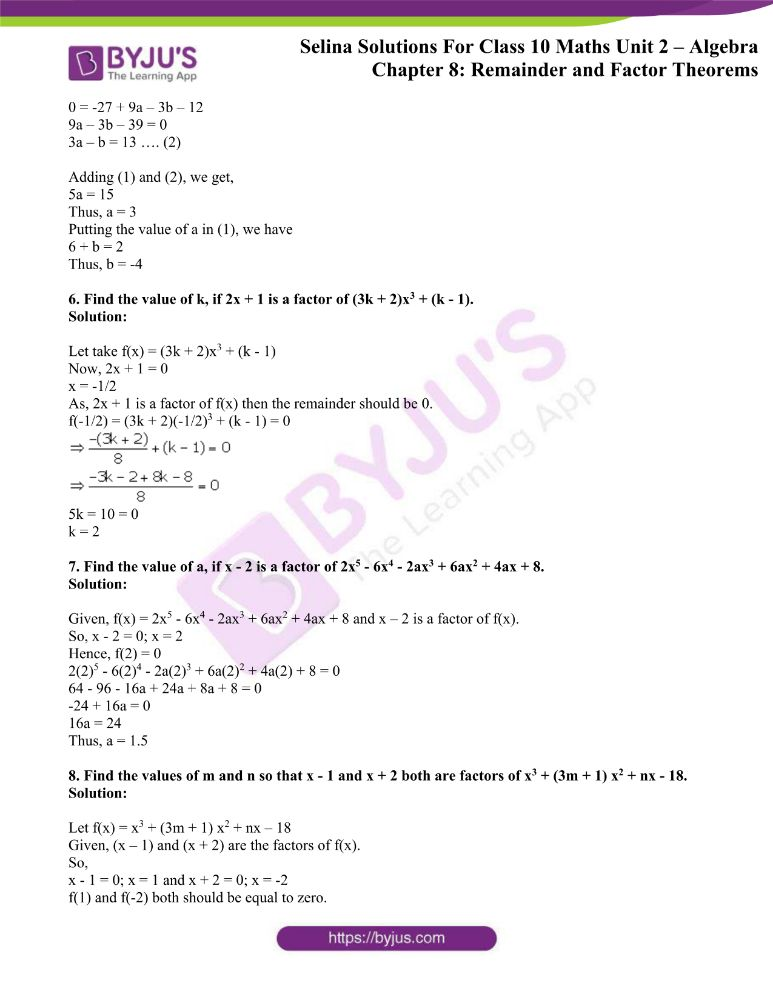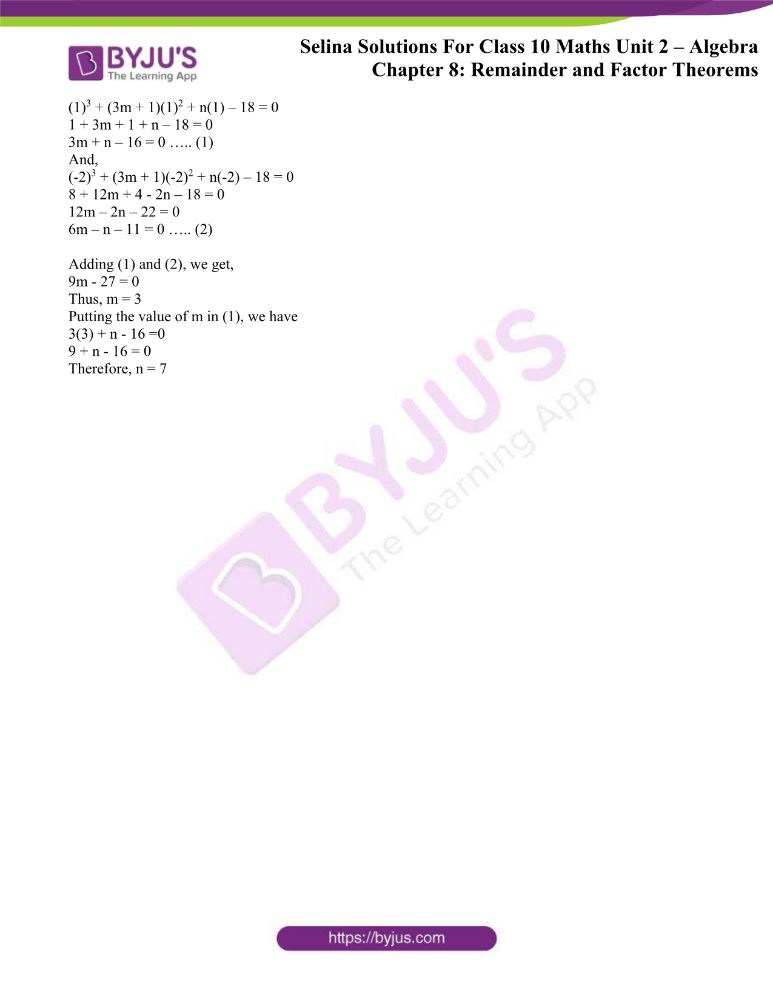# Selina Solutions Concise Maths Class 10 Chapter 8 Remainder and Factor Theorems Exercise 8(A)

Remainder theorem and factor theorem are the two important concepts covered under this exercise. Students wishing to clear their doubts in this and other concepts can utilise the Selina Solutions for Class 10 Maths. The solutions are prepared in a comprehensive manner to make it interesting for students to solve. The links to the solutions of this exercise can be accessed in the Concise Selina Solutions for Class 10 Maths Chapter 8 Remainder and Factor Theorems Exercise 8(A), PDF given below.

## Selina Solutions Concise Maths Class 10 Chapter 8 Remainder and Factor Theorems Exercise 8(A) Download PDF### Access other exercises of Selina Solutions Concise Maths Class 10 Chapter 8 Remainder and Factor Theorems

Exercise 8(B) Solutions

Exercise 8(C) Solutions

### Access Selina Solutions Concise Maths Class 10 Chapter 8 Remainder and Factor Theorems Exercise 8(A)

1. Find, in each case, the remainder when:

(i) x4 – 3x2 + 2x + 1 is divided by x – 1.

(ii) x3 + 3x2 – 12x + 4 is divided by x – 2.

(ii) x4 + 1 is divided by x + 1.

Solution:

From remainder theorem, we know that when a polynomial f (x) is divided by (x – a), then the remainder is f(a).

(i) Given, f(x) = x4 – 3x2 + 2x + 1 is divided by x – 1

So, remainder = f(1) = (1)4 – 3(1)2 + 2(1) + 1 = 1 – 3 + 2 + 1 = 1

(ii) Given, f(x) = x3 + 3x2 – 12x + 4 is divided by x – 2

So, remainder = f(2) = (2)3 + 3(2)2 – 12(2) + 4 = 8 + 12 – 24 + 4 = 0

(iii) Given, f(x) = x4 + 1 is divided by x + 1

So, remainder = f(-1) = (-1)4 + 1 = 2

2. Show that:

(i) x – 2 is a factor of 5x2 + 15x – 50

(ii) 3x + 2 is a factor of 3x2 – x – 2

Solution:

(x – a) is a factor of a polynomial f(x) if the remainder, when f(x) is divided by (x – a), is 0, i.e., if f(a) = 0.

(i) f(x) = 5x2 + 15x – 50

f(2) = 5(2)2 + 15(2) – 50 = 20 + 30 – 50 = 0

As the remainder is zero for x = 2

Thus, we can conclude that (x – 2) is a factor of 5x2 + 15x – 50

(ii) f(x) = 3x2 – x – 2

f(-2/3) = 3(-2/3)2 – (-2/3) – 2 = 4/3 + 2/3 – 2 = 2 – 2 = 0

As the remainder is zero for x = -2/3

Thus, we can conclude that (3x + 2) is a factor of 3x2 – x – 2

3. Use the Remainder Theorem to find which of the following is a factor of 2x3 + 3x2 – 5x – 6.

(i) x + 1 (ii) 2x – 1

(iii) x + 2

Solution:

From remainder theorem we know that when a polynomial f (x) is divided by x – a, then the remainder is f(a).

Here, f(x) = 2x3 + 3x2 – 5x – 6

(i) f (-1) = 2(-1)3 + 3(-1)2 – 5(-1) – 6 = -2 + 3 + 5 – 6 = 0

⇒ Remainder is zero for x = -1

Therefore, (x + 1) is a factor of the polynomial f(x).

(ii) f(1/2) = 2(1/2)3 + 3(1/2)2 – 5(1/2) – 6

= ¼ + ¾ – 5/2 – 6

= -5/2 – 5 = -15/2

⇒ Remainder is not equals to zero for x = 1/2

Therefore, (2x – 1) is not a factor of the polynomial f(x).

(iii) f (-2) = 2(-2)3 + 3(-2)2 – 5(-2) – 6 = -16 + 12 + 10 – 6 = 0

⇒ Remainder is zero for x = -2

Therefore, (x + 2) is a factor of the polynomial f(x).

4. (i) If 2x + 1 is a factor of 2x2 + ax – 3, find the value of a.

(ii) Find the value of k, if 3x – 4 is a factor of expression 3x2 + 2x – k.

Solution:

(i) Given, 2x + 1 is a factor of f(x) = 2x2 + ax – 3.

So, f(-1/2) = 0

2(-1/2)2 + a(-1/2) – 3 = 0

½ – a/2 – 3 = 0

1 – a – 6 = 0

a = -5

(ii) Given, 3x – 4 is a factor of g(x) = 3x2 + 2x – k.

So, f(4/3) = 0

3(4/3)2 + 2(4/3) – k = 0

16/3 + 8/3 – k = 0

24/3 = k

k = 8

5. Find the values of constants a and b when x – 2 and x + 3 both are the factors of expression x3 + ax2 + bx – 12.

Solution:

Here, f(x) = x3 + ax2 + bx – 12

Given, x – 2 and x + 3 both are the factors of f(x)

So,

f(2) and f(-3) both should be equal to zero.

f(2) = (2)3 + a(2)2 + b(2) – 12

0 = 8 + 4a + 2b – 12

0 = 4a + 2b – 4

2a + b = 2 …. (1)

Now,

f(-3) = (-3)3 + a(-3)2 + b(-3) – 12

0 = -27 + 9a – 3b – 12

9a – 3b – 39 = 0

3a – b = 13 …. (2)

Adding (1) and (2), we get,

5a = 15

Thus, a = 3

Putting the value of a in (1), we have

6 + b = 2

Thus, b = -4

6. Find the value of k, if 2x + 1 is a factor of (3k + 2)x3 + (k – 1).

Solution:

Let take f(x) = (3k + 2)x3 + (k – 1)

Now, 2x + 1 = 0

x = -1/2

As, 2x + 1 is a factor of f(x) then the remainder should be 0.

f(-1/2) = (3k + 2)(-1/2)3 + (k – 1) = 05k = 10 = 0

k = 2

7. Find the value of a, if x – 2 is a factor of 2x5 – 6x4 – 2ax3 + 6ax2 + 4ax + 8.

Solution:

Given, f(x) = 2x5 – 6x4 – 2ax3 + 6ax2 + 4ax + 8 and x – 2 is a factor of f(x).

So, x – 2 = 0; x = 2

Hence, f(2) = 0

2(2)5 – 6(2)4 – 2a(2)3 + 6a(2)2 + 4a(2) + 8 = 0

64 – 96 – 16a + 24a + 8a + 8 = 0

-24 + 16a = 0

16a = 24

Thus, a = 1.5

8. Find the values of m and n so that x – 1 and x + 2 both are factors of x3 + (3m + 1) x2 + nx – 18.

Solution:

Let f(x) = x3 + (3m + 1) x2 + nx – 18

Given, (x – 1) and (x + 2) are the factors of f(x).

So,

x – 1 = 0; x = 1 and x + 2 = 0; x = -2

f(1) and f(-2) both should be equal to zero.

(1)3 + (3m + 1)(1)2 + n(1) – 18 = 0

1 + 3m + 1 + n – 18 = 0

3m + n – 16 = 0 ….. (1)

And,

(-2)3 + (3m + 1)(-2)2 + n(-2) – 18 = 0

8 + 12m + 4 – 2n – 18 = 0

12m – 2n – 22 = 0

6m – n – 11 = 0 ….. (2)

Adding (1) and (2), we get,

9m – 27 = 0

Thus, m = 3

Putting the value of m in (1), we have

3(3) + n – 16 =0

9 + n – 16 = 0

Therefore, n = 7